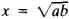# Mean Proportional

Also found in: Dictionary.

## mean proportional

[′mēn prə′pȯr·shən·əl]
(mathematics)
For two numbers a and b, a number x, such that x / a = b / x.
McGraw-Hill Dictionary of Scientific & Technical Terms, 6E, Copyright © 2003 by The McGraw-Hill Companies, Inc.
The following article is from The Great Soviet Encyclopedia (1979). It might be outdated or ideologically biased.

## Mean Proportional

A mean proportional between two positive numbers is a number equal to the square root of their product. Thus, if a:x = x:b, then x is a mean proportional between a and b, and. The mean proportional x is also called the geometric mean of a and b.

The Great Soviet Encyclopedia, 3rd Edition (1970-1979). © 2010 The Gale Group, Inc. All rights reserved.
Mentioned in ?
References in periodicals archive ?
The mean proportional additional error of benefit transfer and its standard error for each activity type are presented in Table 1.
But by electoral reform, I don't mean proportional representation - any MP who is elected should win 50% of the vote.'
Antilogs of the mean differences were calculated to assess the mean proportional biases of the TCEP method with respect to the TBP method.
The mean proportional bias (antilog of the mean difference calculated from log-transformed data) was largest for tCys and tGSH determinations: 65.5% and 59.6%, respectively.
The mean proportional biases between the TBP and TCEP determinations of tCys, tCys-Gly, and tGSH (Table 2) therefore originated in the differences between the aminothiol peak areas in the clinical plasma samples (see above) rather than from the differences between calibration slopes.
Antilogs of the mean differences were calculated to assess the mean proportional bias of each method with respect to GC-MS.
When GC-MS vs FPIA was compared with GC-MS vs HPLC, it became apparent that the above-mentioned ranges of percentages for the two comparisons were virtually the same size for fasting tHcy concentrations, but that HPLC showed a larger negative mean proportional bias (the antilog of the mean difference calculated from log-transformed data) than FPIA.

Site: Follow: Share:
Open / Close